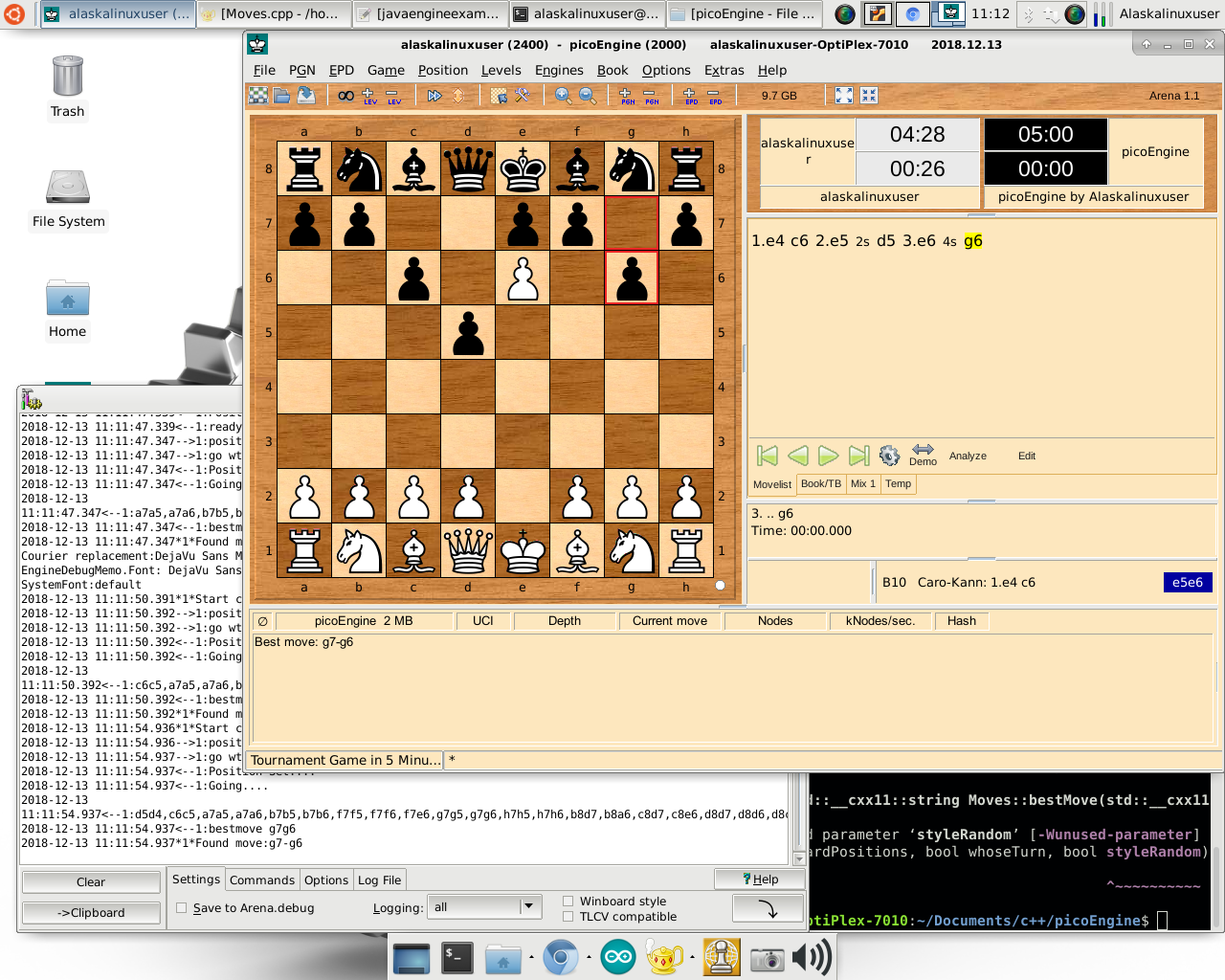Okay, it’s time to work on moves for the last piece: the pawn. Believe it or not, the pawn is the most difficult piece for me to program. It has so many small idiosyncrasies. If you are on the home row, you can go two spaces, but not any other time. If you were just passed by a pawn from it’s home row doing a two step, you can en passant. Oh, and you attack diagonally, but only move directly forward. I think you get the idea.

Just the basic one step, two step, and attack diagonally moves took 50 lines of code for me to write! You can check out the full commit on my GitLab, but here is the important part:

string Moves::pawnMoves(string boardPositions, int i) {
string list = “”;
vector <int> theseMoves;
string moveSquare;
string theBoard = boardPositions;
bool turn = true;
int rowNum = i/8;
int colNum = i%8;
int k = i + 16;
cout << rowNum << endl;
if (rowNum == 1) {
// The standard catch for moving two spaces forward.
if (theBoard[i+8] == ‘-‘ && theBoard[i+16] == ‘-‘) {
theseMoves.push_back(k);
}
}

// The standard catch for moving one space forward.
k = i + 8;
if (theBoard[k] == ‘-‘) {
theseMoves.push_back(k);
}
k = i + 7;// Attacking to the left and up.
if (colNum > 0 && islower(theBoard[k])) {
theseMoves.push_back(k);
}
k = i + 9;// Attacking to the right and up.
if (colNum < 7 && islower(theBoard[k])) {
theseMoves.push_back(k);
}
// End boring pawn moves.

// Need en passant moves //

for(int l=0; (unsigned)l<theseMoves.size();l++) {
int k = theseMoves[l];
if (islower(theBoard[k]) || theBoard[k] == ‘-‘) {
moveSquare = theBoard[k];
theBoard[k] = ‘P’;
theBoard[i] = ‘-‘;
if (isKingSafe(theBoard, turn)) {
char F = (char)(‘a’ + colNum);
char G = (char)(‘1’ + rowNum);
int rowNumK = k/8;
int colNumK = k%8;
char T = (char)(‘a’ + colNumK);
char U = (char)(‘1’ + rowNumK);
list = list + F + G + T + U + “,”;
}
theBoard[k] = moveSquare;
theBoard[i] = ‘P’;
}
}
return list;
} // End white pawn moves.

Now I just have to work on en passant, and work on the king’s castle moves. Almost done with moves so I can actually focus on building the “thinking” part of the engine!

Linux – keep it simple.